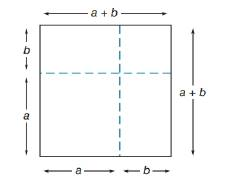Chapter 8.1, Problem 46E### Elementary Geometry for College St...

6th Edition
Daniel C. Alexander + 1 other
ISBN: 9781285195698

#### Solutions

Chapter
Section### Elementary Geometry for College St...

6th Edition
Daniel C. Alexander + 1 other
ISBN: 9781285195698
Textbook Problem
1 views

# Use the square configuration to complete the following algebra rule: ( a + b ) 2 = _ _ _ _ _ _ _ _ _ _ _ _ _ _ _ _ _ _ _ _ _ _ (NOTE: Simplify where possible.)To determine

To complete:

(a+b)2=_ by the square configuration of the drawing.

Explanation

Multiplication of the terms is followed by the FOIL method,

Calculation:

Given,

By the drawing which is a square whose side length s=(a+b) and width w=(c+d)

The area of the square =A=(s)2unit2.

A=(a+b)2

A=(a+b)(a+b)

By the FOIL method,

=aa+ba+ab+bb

=a2+ba+ab+b2.

Since, the multiplication is commutative.

(i.e

### Still sussing out bartleby?

Check out a sample textbook solution.

See a sample solution

#### The Solution to Your Study Problems

Bartleby provides explanations to thousands of textbook problems written by our experts, many with advanced degrees!

Get Started

#### Find more solutions based on key concepts